### Response Level Ordering

Response level ordering is important because, by default, PROC LOGISTIC models the probability of response levels with lower Ordered Value. Ordered Values are assigned to response levels in ascending sorted order (that is, the lowest response level is assigned Ordered Value 1, the next lowest is assigned Ordered Value 2, and so on) and are displayed in the Response Profiles table. If your response variable Y takes values in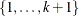, then, by default, the functions modeled with the binary or cumulative model are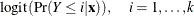and for the generalized logit model the functions modeled are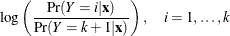where the highest Ordered Value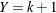is the reference level. You can change which probabilities are modeled by specifying the EVENT=, REF=, DESCENDING, or ORDER= response variable options in the MODEL statement.

For binary response data with event and nonevent categories, if your event category has a higher Ordered Value, then by default the nonevent is modeled. Since the default response function modeled is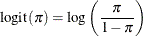where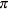is the probability of the response level assigned Ordered Value 1, and since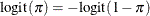the effect of modeling the nonevent is to change the signs ofandin the model for the event,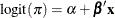.

For example, suppose the binary response variable Y takes the values 1 and 0 for event and nonevent, respectively, and Exposure is the explanatory variable. By default, PROC LOGISTIC assigns Ordered Value 1 to response level Y=0, and Ordered Value 2 to response level Y=1. As a result, PROC LOGISTIC models the probability of the nonevent (Ordered Value=1) category, and your parameter estimates have the opposite sign from those in the model for the event. To model the event without using a DATA step to change the values of the variable Y, you can control the ordering of the response levels or select the event or reference level, as shown in the following list:

• Explicitly state which response level is to be modeled by using the response variable option EVENT= in the MODEL statement:

   model Y(event='1') = Exposure;

• Specify the nonevent category for the response variable in the response variable option REF= in the MODEL statement. This option is most useful for generalized logit models where the EVENT= option cannot be used.

   model Y(ref='0') = Exposure;

• Specify the response variable option DESCENDING in the MODEL statement to assign the lowest Ordered Value to Y=1:

   model Y(descending)=Exposure;

• Assign a format to Y such that the first formatted value (when the formatted values are put in sorted order) corresponds to the event. In the following example, Y=1 is assigned the formatted value ‘event’ and Y=0 is assigned the formatted value ‘nonevent’. Since ORDER=FORMATTED by default, Ordered Value 1 is assigned to response level Y=1, so the procedure models the event.

proc format;
value Disease 1='event' 0='nonevent';
run;
proc logistic;
format Y Disease.;
model Y=Exposure;
run;